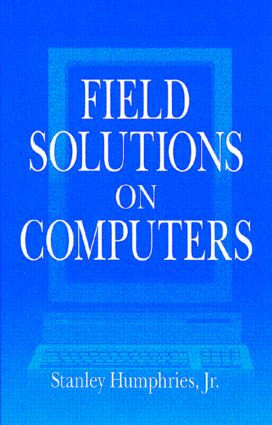# Field Solutions on Computers

## 1st Edition

CRC Press

304 pages

##### Purchasing Options:\$ = USD
Hardback: 9780849316685
pub: 1997-12-29
\$185.00
x

FREE Standard Shipping!

### Description

Field Solutions on Computers covers a broad range of practical applications involving electric and magnetic fields. The text emphasizes finite-element techniques to solve real-world problems in research and industry. After introducing numerical methods with a thorough treatment of electrostatics, the book moves in a structured sequence to advanced topics. These include magnetostatics with non-linear materials, permanent magnet devices, RF heating, eddy current analysis, electromagnetic pulses, microwave structures, and wave scattering. The mathematical derivations are supplemented with chapter exercises and comprehensive reviews of the underlying physics. The book also covers essential supporting techniques such as mesh generation, interpolation, sparse matrix inversions, and advanced plotting routines.

Introduction

Overview

Some Precautions

Finite-Element Electrostatic Equations

Coulomb's Law

Gauss's Law and Charge Density

Differential Equations for Electrostatic Fields

Charge Density Distributions and Dielectric Materials

Finite Elements

Coordinate Relationships for Triangles

Gauss's Law for Elements at a Vertex Point

Solution Procedure and Boundary Conditions

Electrostatics in Cylindrical Coordinates

Exercises

Minimum-Energy Principles in Electrostatics

Electrostatic Field Energy

Elements of the Calculus of Variations

Poisson Equation As a Condition of Minimum Energy

Finite-Element Equations for Two-Dimensional Electrostatics

Three-Dimensional Finite-Element Electrostatics on Arbitrary Meshes

Higher-Order Finite-Element Formulations

Exercises

Finite-Difference Solutions and Regular Meshes

Difference Operators

Initial Value Solutions of Ordinary Differential Equations

One-Dimensional Poisson Equation

Solution of the Poisson Equation by Back-Substitution

Two-Dimensional Electrostatic Solutions on a Regular Mesh

Three-Dimensional Electrostatic Solutions on a Regular Mesh

Exercises

Techniques for Numerical Field Solutions

Regular Meshes in Three Dimensions

Two-Dimensional Conformal Triangular Meshes

Fitting Triangular Elements to Physical Boundaries

Neumann Boundaries in Resistive Media

Boundary Value solutions by Successive Over-Relaxation

Exercises

Matrix Methods for Field Solutions

Introduction

Gauss-Jordan Elimination

Solving Tridiagonal Matrices

Matrix Solutions for One-Dimensional Electrostatics

Matrices for Two-Dimensional Finite-Element Solutions

Solving Tridiagonal Block Matrix Problems

Exercises

Analyzing Numerical Solutions

Locating Elements

Generalized Least-Square Fits

Field Calculations on a Two-Dimensional Triangular Mesh

Mesh and Boundary Plots

Contour, Element, Elevation, and Field Line Plots

Exercises

Nonlinear and Anisotropic Materials

Iterative Solutions to Boundary Value Problems

Numerical Data for Material Properties

Finite-Element Equations for Anisotropic Materials

Exercises

Finite-Element Magnetostatic Solutions

Differential and Integral Magnetostatic Equations

Vector Potential and Field Equations in Two Dimensions

Isotropic Magnetic Materials

Finite-Element Magnetostatic Equations

Magnetic Field Solutions

Properties of Permanent Magnet Materials

Magnetostatic Solutions with Permanent Magnets

Exercises

Static Field Analysis and Applications

Volume and Surface Integrals on a Finite-Element Mesh

Electric and Magnetic Field Energy

Capacitance Calculations

Inductance Calculations

Electric and Magnetic Forces on Materials

Charged-Particle Orbits

Electron and Ion Guns

Generalized Neumann Boundaries - Hall Effect Devices

Exercises

Low-Frequency Electric and Magnetic Fields

Maxwell Equations

Complex Numbers for Harmonic Quantities

Electric Field Equations in Resistive Media

Electric Field Solutions with Complex Number Potentials

Magnetic Fields with Eddy Currents

Exercises

Thermal Transport and Magnetic Field Diffusion

Thermal Transport Equation

Finite-Difference Solution of the Diffusion Equation

Finite-Element Diffusion Solutions

Instabilities in Finite-Element Diffusion Solutions

Magnetic Field Diffusion

Exercises

Electromagnetic Fields in One Dimension

Planar Electromagnetic Waves

Time-Domain Electromagnetism in One Dimension

Electromagnetic Pulse Solutions

Frequency-Domain Equations

Scattering Solutions

One-Dimensional Resonant Modes

Exercises

Two- and Three-Dimensional Electromagnetic Simulations

Introduction

Time-Domain Equations on a Conformal Mesh

Electromagnetic Pulse Solutions

Frequency-Domain Equations

Methods for Scattering Solutions

Waveguides and Resonant Cavities

Power Losses and Q Factors

Finite-Difference Time-Domain Method in Three Dimensions

Three-Dimensional Element-Based Time-Domain Equations

Exercises

Bibliography

Index

### Subject Categories

##### BISAC Subject Codes/Headings:
MAT000000
MATHEMATICS / General
TEC007000
TECHNOLOGY & ENGINEERING / Electrical